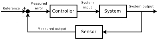xControl theoryOverview

Control theory is an interdisciplinary branch of engineering
Engineering
Engineering is the discipline, art, skill and profession of acquiring and applying scientific, mathematical, economic, social, and practical knowledge, in order to design and build structures, machines, devices, systems, materials and processes that safely realize improvements to the lives of...

and mathematics
Mathematics
Mathematics is the study of quantity, space, structure, and change. Mathematicians seek out patterns and formulate new conjectures. Mathematicians resolve the truth or falsity of conjectures by mathematical proofs, which are arguments sufficient to convince other mathematicians of their validity...

that deals with the behavior of dynamical system
Dynamical system
A dynamical system is a concept in mathematics where a fixed rule describes the time dependence of a point in a geometrical space. Examples include the mathematical models that describe the swinging of a clock pendulum, the flow of water in a pipe, and the number of fish each springtime in a...

s. The desired output of a system is called the reference
Reference
Reference is derived from Middle English referren, from Middle French rèférer, from Latin referre, "to carry back", formed from the prefix re- and ferre, "to bear"...

. When one or more output variables of a system need to follow a certain reference over time, a controller
Controller (control theory)
In control theory, a controller is a device which monitors and affects the operational conditions of a given dynamical system. The operational conditions are typically referred to as output variables of the system which can be affected by adjusting certain input variables...

manipulates the inputs to a system to obtain the desired effect on the output of the system.

The usual objective of control theory is to calculate solutions for the proper corrective action from the controller that result in system stability, that is, the system will hold the set point and not oscillate around it.

The input and ouput of the system are related to each other by what is known as a transfer function
Transfer function
A transfer function is a mathematical representation, in terms of spatial or temporal frequency, of the relation between the input and output of a linear time-invariant system. With optical imaging devices, for example, it is the Fourier transform of the point spread function i.e...

(also known as the system function or network function).